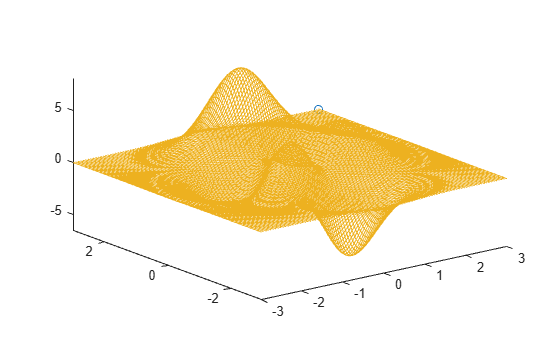# comet3

3-D comet plot

## Syntax

``comet3(z)``
``comet3(x,y,z)``
``comet3(x,y,z,p)``
``comet3(ax,x,y,z,p)``

## Description

````comet3(z)` displays a comet plot of the data specified in `z` versus `x`- and `y`-values matching the indices of `z`. A comet is an animation of a marker (head) and a line (tail) tracing a growing line over the data points. The tail is a solid line that traces the entire function.```

example

````comet3(x,y,z)` displays a comet plot of `z` versus `x` and `y`.```

example

````comet3(x,y,z,p)` specifies the comet body length. The comet body is a trailing segment in a different color that follows the head before fading. The body length is `p*length(y)`, where p is a scalar in [0, 1).```

example

````comet3(ax,x,y,z,p)` plots the comet into the axes specified by `ax`.```

## Examples

collapse all

Create a comet plot of the data in `z` versus the data in `x` and `y`. Use the `peaks` function to load `x`, `y`, and `z` data in matrix forms. Convert the data into vector arrays. Then, plot the data.

```[xmat,ymat,zmat] = peaks(100); xvec = xmat(:); yvec = ymat(:); zvec = zmat(:); comet3(xvec,yvec,zvec)```Create a comet plot and specify the comet body length by setting the scale factor input `p`. The comet body is a trailing segment in a different color that follows the head before fading.

Use the `peaks` function to load `x`, `y`, and `z` data in matrix forms. Convert the data into vector arrays. Specify `p` as `0.5` so that the body length is `0.5*length(y)`. Then, plot the data.

```[xmat,ymat,zmat] = peaks(100); xvec = xmat(:); yvec = ymat(:); zvec = zmat(:); p = 0.5; comet3(xvec,yvec,zvec,p)```Create two comet plots in a tiled chart layout by specifying the target axes for each plot.

Use the `peaks` function to load `x`, `y`, and `z` data in matrix forms. Convert the data into vector arrays. Specify the body length scale factor `p` as `0.25` so that the body length is `0.5*length(y)`.

```[xmat,ymat,zmat] = peaks(50); xvec = xmat(:); yvec = ymat(:); zvec = zmat(:); p = 0.25;```

Store the two `Axes` objects as `ax1` and `ax2`. Specify the target axes for each comet plot by including the `Axes` object as the first input argument to `comet`.

```tiledlayout(1,2); ax1 = nexttile; ax2 = nexttile; comet3(ax1,xvec,yvec,zvec,p) comet3(ax2,yvec,xvec,zvec,p)```## Input Arguments

collapse all

z-values, specified as a vector. To plot against specific x- and y-values you must also specify `x` and `y`.

x-values, specified as a vector. `x`, `y`, and `z` must be the same size. If you specify geographic axes for `ax`, then `x` represents latitude in degrees.

y-values, sp0ecified as a vector. `x`, `y`, and `z` must be the same size. If you specify geographic axes for `ax`, then `y` represents longitude in degrees.

Comet body length scale factor, specified as a value in the interval [0, 1). The comet body length is `p*length(y)`.

Target axes, specified as an `Axes` object or `GeographicAxes` object.

## Limitations

• Comet plots do not support data tips.

## Version History

Introduced before R2006a